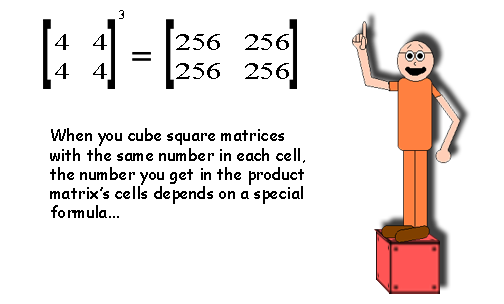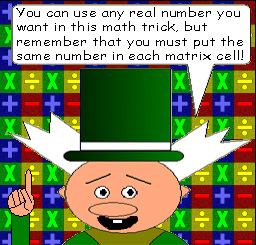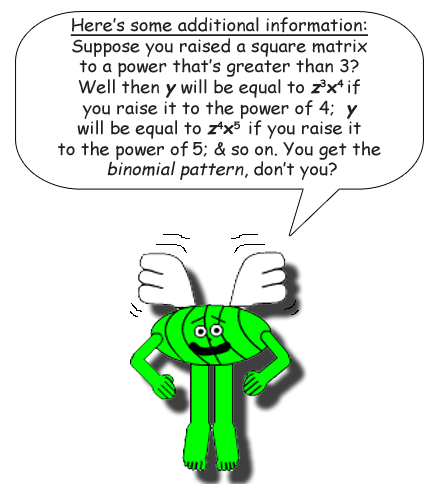# Cubing Square MatricesDo you see the pattern yet with the matrices on the bottom row above? The irrational number pi is in each cell of those matrices to give you a clue! If you still don't get it, the function can be found just below this paragraph!

## There's a function for this math trick. The function is:

y = z2x3

x = the number in each matrix cell

y = the number of rows & columns squared multiplied by the cube of the number in each matrix cell

z = the number of rows & columns

## Remember Math Trick #12: "Squaring Square Matrices"? Well the formula for that trick is multiplied by the product of the 2 variables z & x, which is zx! In other words, zx2 * zx = z2x3